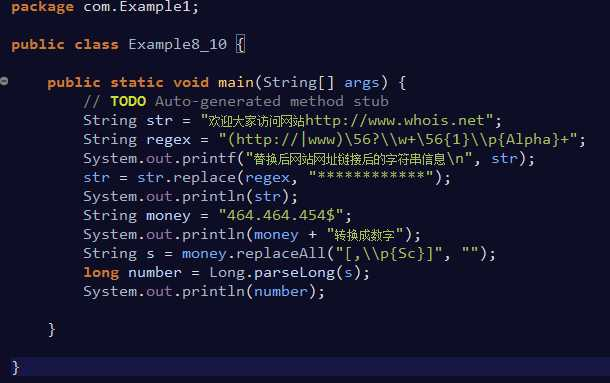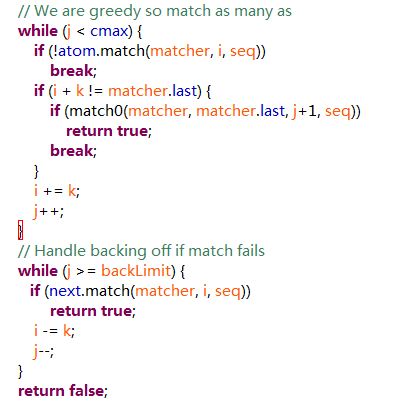# 深入浅解正则表达式在Java中的使用_正则表达式

## 介绍

•正则表达式一般用于字符串匹配, 字符串查找和字符串替换. 别小看它的作用, 在工作学习中灵活运用正则表达式处理字符串能够大幅度提高效率, 编程的快乐来得就是这么简单.

String regex = "\\(.*)\\\\s+\\\\s+\\(.*)\\s+(.*)\\";•一下子给出一堆匹配的规则可能会让人恐惧, 下面将由浅入深讲解正则表达式的使用.

## 从简单例子认识正则表达式匹配比如有正则表达式如下: "\d+"和"^\d+" 那么字符串"a123456789"匹配第一个正则表达式是能匹配通过的,但是第二个就不行 ^符号在正则表达式第一位出现表示的是强匹配开头,也就是必须是\d+开头的字符串才能匹配通过,中间一部分符合\d+规则的匹配不通•先上代码public class Demo1 { public static void main(String[] args) { //字符串abc匹配正则表达式"...", 其中"."表示一个字符 //"..."表示三个字符 System.out.println("abc".matches("...")); System.out.println("abcd".matches("...")); }}//输出结果truefalse

import java.util.regex.Matcher;import java.util.regex.Pattern;public class JButtonTest{public static void main ( String[] args ){String input = "abcdd[a-b]df[3.b]";String regex = "\\[[^\\[\\]]*\\]";Pattern pattern = Pattern.•String类中有个`matches(String regex)`方法, 返回值为布尔类型, 用于告诉这个字符串是否匹配给定的正则表达式.

•在本例中我们给出的正则表达式为..., 其中每个.表示一个字符, 整个正则表达式的意思是三个字符, 显然当匹配abc的时候结果为true, 匹配abcd时结果为false.

## Java中对正则表达式的支持(各种语言有相应的实现)

•在java.util.regex包下有两个用于正则表达式的类, 一个是Matcher类, 另一个Pattern. Java官方文档中给出对这两个类的典型用法, 代码如下:public class Demo1 { public static void main(String[] args) { //字符串abc匹配正则表达式"...", 其中"."表示一个字符 //"..."表示三个字符 System.out.println("abc".matches("...")); System.out.println("abcd".matches("...")); }}//输出结果truefalse

import java.util.regex.Matcher;import java.util.regex.Pattern;public class JButtonTest{public static void main ( String[] args ){String input = "abcdd[a-b]df[3.b]";String regex = "\\[[^\\[\\]]*\\]";Pattern pattern = Pattern.•如果要深究正则表达式背后的原理, 会涉及编译原理中自动机等知识, 此处不展开描述. 为了达到通俗易懂, 这里用较为形象的语言描述.

•Pattern可以理解为一个模式, 字符串需要与某种模式进行匹配. 比如Demo2中, 我们定义的模式是一个长度为3的字符串, 其中每个字符必须是a~z中的一个.

•我们看到创建Pattern对象时调用的是Pattern类中的compile方法, 也就是说对我们传入的正则表达式编译后得到一个模式对象. 而这个经过编译后模式对象, 会使得正则表达式使用效率会大大提高, 并且作为一个常量, 它可以安全地供多个线程并发使用.

•Matcher可以理解为模式匹配某个字符串后产生的结果. 字符串和某个模式匹配后可能会产生很多个结果, 这个会在后面的例子中讲解.

•最后当我们调用m.matches()时就会返回完整字符串与模式匹配的结果

•上面的三行代码可以简化为一行代码

`System.out.println("abc".matches("[a-z]{3}"));`

•但是如果一个正则表达式需要被重复匹配, 这种写法效率较低.

## 初步认识 . + * ?

•在介绍之前首先要说明的是, 正则表达式的具体含义不用强背, 各个符号的含义在Java官方文档的Pattern类描述中或网上有详细的定义. 当然能熟用就更好了.public class Demo3 { /** * 为了省略每次写打印语句, 这里把输出语句封装起来 * @param o */ private static void p(Object o){ System.out.println(o); } /** * . Any character (may or may not match line terminators), 任意字符 * X? X, once or not at all 零个或一个 * X* X, zero or more times 零个或多个 * X+ X, one or more times 一个或多个 * X{n} X, exactly n times x出现n次 * X{n,} X, at least n times x出现至少n次 * X{n,m} X, at least n but not more than m times 出现n~m次 * @param args */ public static void main(String[] args) { p("a".matches(".")); p("aa".matches("aa")); p("aaaa".matches("a*")); p("aaaa".matches("a+")); p("".matches("a*")); p("a".matches("a?")); // \d A digit: [0-9], 表示数字, 但是在java中对"\"这个符号需要使用\进行转义, 所以出现\\d p("2345".matches("\\d{2,5}")); // \\.用于匹配"." p("192.168.0.123".matches("\\d{1,3}\\.\\d{1,3}\\.\\d{1,3}\\.\\d{1,3}")); // [0-2]指必须是0~2中的一个数字 p("192".matches("[0-2][0-9][0-9]")); }}//输出结果//全为true

## 范围

•[]用于描述一个字符的范围, 下面是一些例子public class Demo4 { private static void p(Object o){ System.out.println(o); } public static void main(String[] args) { //[abc]指abc中的其中一个字母 p("a".matches("[abc]")); //[^abc]指除了abc之外的字符 p("1".matches("[^abc]")); //a~z或A~Z的字符, 以下三个均是或的写法 p("A".matches("[a-zA-Z]")); p("A".matches("[a-z|A-Z]")); p("A".matches("[a-z[A-Z]]")); //[A-Z&&[REQ]]指A~Z中并且属于REQ其中之一的字符 p("R".matches("[A-Z&&[REQ]]")); }}//输出结果

## 全部为true

•这里重点介绍最不好理解的\. 在Java中的字符串中, 如果要用到特殊字符, 必须通过在前面加\进行转义.

•举个例子, 考虑这个字符串"老师大声说:"同学们,快交作业!"". 如果我们没有转义字符, 那么开头的双引号的结束应该在说:"这里, 但是我们的字符串中需要用到双引号, 所以需要用转义字符

•使用转义字符后的字符串为"老师大声说:\"同学们,快交作业!\"", 这样我们的原意才能被正确识别.

•同理如果我们要在字符串中使用\, 也应该在前面加一个\, 所以在字符串中表示为"\\"

•那么如何在正则表达式中表示要匹配\呢, 答案为"\\\\".

•我们分开考虑: 由于正则式中表示\同样需要转义, 所以前面的\\表示正则表达式中的转义字符\, 后面的\\表示正则表达式中\本身, 合起来在正则表达式中表示\.

•如果感觉有点绕的话请看下面代码public class Demo5 { private static void p(Object o){ System.out.println(o); } public static void main(String[] args) { /** * \d A digit: [0-9] 数字 * \D A non-digit: [^0-9] 非数字 * \s A whitespace character: [ \t\n\x0B\f\r] 空格 * \S A non-whitespace character: [^\s] 非空格 * \w A word character: [a-zA-Z_0-9] 数字字母和下划线 * \W A non-word character: [^\w] 非数字字母和下划线 */ // \\s{4}表示4个空白符 p(" \n\r\t".matches("\\s{4}")); // \\S表示非空白符 p("a".matches("\\S")); // \\w{3}表示数字字母和下划线 p("a_8".matches("\\w{3}")); p("abc888&^%".matches("[a-z]{1,3}\\d+[%^&*]+")); // 匹配 \ p("\\".matches("\\\\")); }}//输出结果

## 全部为true

•^在中括号内表示取反的意思[^], 如果不在中括号里则表示字符串的开头.public class Demo6 { private static void p(Object o){ System.out.println(o); } public static void main(String[] args) { /** * ^ The beginning of a line 一个字符串的开始 * \$ The end of a line 字符串的结束 * \b A word boundary 一个单词的边界, 可以是空格, 换行符等 */ p("hello sir".matches("^h.*")); p("hello sir".matches(".*r\$")); p("hello sir".matches("^h[a-z]{1,3}o\\b.*")); p("hellosir".matches("^h[a-z]{1,3}o\\b.*")); }}

## 练习:匹配空白行合email地址

•拿到一篇文章, 如何判断里面有多少个空白行? 用正则表达式能方便地进行匹配, 注意空白行中可能包括空格, 制表符等.

p(" \n".matches("^[\\s&&[^\n]]*\\n\$"));

•解释: ^[\\s&&[^\n]]*是空格符号但不是换行符, \\n\$最后以换行符结束

•下面是匹配邮箱

•解释: [\\w[.-]]+以一个或多个数字字母下划线.或-组成, @接着是个@符号, 然后同样是[\\w[.-]]+, 接着\\.匹配., 最后同样是[\\w]+

Matcher类的matches(),find()和lookingAt()

•matches()方法会将整个字符串与模板进行匹配.

•find()则是从当前位置开始进行匹配, 如果传入字符串后首先进行find(), 那么当前位置就是字符串的开头, 对当前位置的具体分析可以看下面的代码示例

•lookingAt()方法会从字符串的开头进行匹配.public class Demo8 { private static void p(Object o){ System.out.println(o); } public static void main(String[] args) { Pattern pattern = Pattern.compile("\\d{3,5}"); String s = "123-34345-234-00"; Matcher m = pattern.matcher(s); //先演示matches(), 与整个字符串匹配. p(m.matches()); //结果为false, 显然要匹配3~5个数字会在-处匹配失败 //然后演示find(), 先使用reset()方法把当前位置设置为字符串的开头 m.reset(); p(m.find());//true 匹配123成功 p(m.find());//true 匹配34345成功 p(m.find());//true 匹配234成功 p(m.find());//false 匹配00失败 //下面我们演示不在matches()使用reset(), 看看当前位置的变化 m.reset();//先重置 p(m.matches());//false 匹配整个字符串失败, 当前位置来到- p(m.find());// true 匹配34345成功 p(m.find());// true 匹配234成功 p(m.find());// false 匹配00始边 p(m.find());// false 没有东西匹配, 失败 //演示lookingAt(), 从头开始找 p(m.lookingAt());//true 找到123, 成功 }}

## Matcher类中的start()和end()

•如果一次匹配成功的话start()用于返回匹配开始的位置, end()用于返回匹配结束字符的后面一个位置public class Demo9 { private static void p(Object o){ System.out.println(o); } public static void main(String[] args) { Pattern pattern = Pattern.compile("\\d{3,5}"); String s = "123-34345-234-00"; Matcher m = pattern.matcher(s); p(m.find());//true 匹配123成功 p("start: " + m.start() + " - end:" + m.end()); p(m.find());//true 匹配34345成功 p("start: " + m.start() + " - end:" + m.end()); p(m.find());//true 匹配234成功 p("start: " + m.start() + " - end:" + m.end()); p(m.find());//false 匹配00失败 try { p("start: " + m.start() + " - end:" + m.end()); }catch (Exception e){ System.out.println("报错了..."); } p(m.lookingAt()); p("start: " + m.start() + " - end:" + m.end()); }}//输出结果truestart: 0 - end:3truestart: 4 - end:9truestart: 10 - end:13false报错了...truestart: 0 - end:3

•想要替换字符串首先要找到被替换的字符串, 这里要新介绍Matcher类中的一个方法group(), 它能返回匹配到的字符串.

•下面我们看一个例子, 把字符串中的java转换为大写.public class Demo10 { private static void p(Object o){ System.out.println(o); } public static void main(String[] args) { Pattern p = Pattern.compile("java"); Matcher m = p.matcher("java Java JAVA JAva I love Java and you"); p(m.replaceAll("JAVA"));//replaceAll()方法会替换所有匹配到的字符串 }}//输出结果JAVA Java JAVA JAva I love Java and you•为了在匹配的时候不区分大小写, 我们要在创建模板模板时指定大小写不敏感public static void main(String[] args) { Pattern p = Pattern.compile("java", Pattern.CASE_INSENSITIVE);//指定为大小写不敏感的 Matcher m = p.matcher("java Java JAVA JAva I love Java and you"); p(m.replaceAll("JAVA"));}//输出结果JAVA JAVA JAVA JAVA I love JAVA and you

## 再升级: 不区分大小写, 替换查找到的指定字符串

•这里演示把查找到第奇数个字符串转换为大写, 第偶数个转换为小写

•这里会引入Matcher类中一个强大的方法appendReplacement(StringBuffer sb, String replacement), 它需要传入一个

StringBuffer进行字符串拼接.public static void main(String[] args) { Pattern p = Pattern.compile("java", Pattern.CASE_INSENSITIVE); Matcher m = p.matcher("java Java JAVA JAva I love Java and you ?"); StringBuffer sb = new StringBuffer(); int index = 1; while(m.find()){ //m.appendReplacement(sb, (index++ & 1) == 0 ? "java" : "JAVA"); 较为简洁的写法 if((index & 1) == 0){//偶数 m.appendReplacement(sb, "java"); }else{ m.appendReplacement(sb, "JAVA"); } index++; } m.appendTail(sb);//把剩余的字符串加入 p(sb);}//输出结果JAVA java JAVA java I love JAVA and you ?

## 分组

•先从一个问题引入, 看下面这段代码public static void main(String[] args) { Pattern p = Pattern.compile("\\d{3,5}[a-z]{2}"); String s = "123aa-5423zx-642oi-00"; Matcher m = p.matcher(s); while(m.find()){ p(m.group()); }}//输出结果123aa5423zx642oi

•其中正则表达式"\\d{3,5}[a-z]{2}"表示3~5个数字跟上两个字母, 然后打印出每个匹配到的字符串.

•如果想要打印每个匹配串中的数字, 如何操作呢.

•首先你可能想到把匹配到的字符串再进行匹配, 但是这样太麻烦了, 分组机制可以帮助我们在正则表达式中进行分组.

•规定使用()进行分组, 这里我们把字母和数字各分为一组"(\\d{3,5})([a-z]{2})"

•然后在调用m.group(int group)方法时传入组号即可

•注意, 组号从0开始, 0组代表整个正则表达式, 从0之后, 就是在正则表达式中从左到右每一个左括号对应一个组. 在这个表达式中第1组是数字, 第2组是字母.public static void main(String[] args) { Pattern p = Pattern.compile("(\\d{3,5})([a-z]{2})");//正则表达式为3~5个数字跟上两个字母 String s = "123aa-5423zx-642oi-00"; Matcher m = p.matcher(s); while(m.find()){ p(m.group(1)); }}//输出结果1235423642

## 实战1: 抓取网页中的email地址(爬虫)

•假设我们手头上有一些优质的资源, 打算分享给网友, 于是便到贴吧上发出一个留邮箱发资源的帖子. 没想到网友热情高涨, 留下了近百个邮箱. 但逐个复制发送太累了, 我们考虑用程序实现.

•这里不展开讲发邮件部分, 重点应用已经学到的正则表达式从网页中截取所有的邮箱地址.

•首先获取一个帖子的html代码随便找了一个, 点击跳转, 在浏览器中点击右键保存html文件

•接下来看代码:public class Demo12 { public static void main(String[] args) { BufferedReader br = null; try { br = new BufferedReader(new FileReader("C:\\emailTest.html")); String line = ""; while((line = br.readLine()) != null){//读取文件的每一行 parse(line);//解析其中的email地址 } } catch (FileNotFoundException e) { e.printStackTrace(); } catch (IOException e) { e.printStackTrace(); }finally { if(br != null){ try { br.close(); br = null; } catch (IOException e) { e.printStackTrace(); } } } } private static void parse(String line){ Pattern p = Pattern.compile("[\\w[.-]]+@[\\w[.-]]+\\.[\\w]+"); Matcher m = p.matcher(line); while(m.find()){ System.out.println(m.group()); } }}//输出结果2819531636@qq.com2819531636@qq.com2405059759@qq.com2405059759@qq.com1013376804@qq.com...

## 实战2: 代码统计小程序

•最后的一个实战案例: 统计一个项目中一共有多少行代码, 多少行注释, 多少个空白行. 不妨对自己做过的项目进行统计, 发现不知不觉中也是个写过成千上万行代码的人了...

•我在github上挑选了一个项目, 是纯java写的小项目, 方便统计. 点击跳转

•下面是具体的代码, 除了判断空行用了正则表达式外, 判断代码行和注释行用了String类的apipublic class Demo13 { private static long codeLines = 0; private static long commentLines = 0; private static long whiteLines = 0; private static String filePath = "C:\\TankOnline"; public static void main(String[] args) { process(filePath); System.out.println("codeLines : " + codeLines); System.out.println("commentLines : " + commentLines); System.out.println("whiteLines : " + whiteLines); } /** * 递归查找文件 * @param pathStr */ public static void process(String pathStr){ File file = new File(pathStr); if(file.isDirectory()){//是文件夹则递归查找 File[] fileList = file.listFiles(); for(File f : fileList){ String fPath = f.getAbsolutePath(); process(fPath); } }else if(file.isFile()){//是文件则判断是否是.java文件 if(file.getName().matches(".*\\.java\$")){ parse(file); } } } private static void parse(File file) { BufferedReader br = null; try { br = new BufferedReader(new FileReader(file)); String line = ""; while((line = br.readLine()) != null){ line = line.trim();//清空每行首尾的空格 if(line.matches("^[\\s&&[^\\n]]*\$")){//注意不是以\n结尾, 因为在br.readLine()会去掉\n whiteLines++; }else if(line.startsWith("/*") || line.startsWith("*") || line.endsWith("*/")){ commentLines++; }else if(line.startsWith("//") || line.contains("//")){ commentLines++; }else{ if(line.startsWith("import") || line.startsWith("package")){//导包不算 continue; } codeLines++; } } } catch (FileNotFoundException e) { e.printStackTrace(); } catch (IOException e) { e.printStackTrace(); } finally { if(null != br){ try { br.close(); br = null; } catch (IOException e) { e.printStackTrace(); } } } }}//输出结果codeLines : 1139commentLines : 124whiteLines : 172

## 贪婪模式与非贪婪模式

•经过两个实战后, 相信大家已经掌握了正则表达式的基本使用了, 下面介绍贪婪模式与非贪婪模式. 通过查看官方api我们发现Pattern类中有如下定义:

## Greedy quantifiers 贪婪模式

X?  X, once or not at all

X*  X, zero or more times

X+  X, one or more times

X{n}    X, exactly n times

X{n,}   X, at least n times

X{n,m}  X, at least n but not more than m times

## Reluctant quantifiers 非贪婪模式(勉强的, 不情愿的)

X?? X, once or not at all

X*? X, zero or more times

X+? X, one or more times

X{n}?   X, exactly n times

X{n,}?  X, at least n times

X{n,m}? X, at least n but not more than m times

## Possessive quantifiers  独占模式

X?+ X, once or not at all

X*+ X, zero or more times

X++ X, one or more times

X{n}+   X, exactly n times

X{n,}+  X, at least n times

X{n,m}+ X, at least n but not more than m times

•这三种模式表达的意思是一样的, 在前面的讲解中我们全部使用的是贪婪模式. 那么其他两种模式的写法有什么区别呢? 通过下面的代码示例进行讲解.

public static void main(String[] args) { Pattern p = Pattern.compile(".{3,10}[0-9]"); String s = "aaaa5bbbb6";//10个字符 Matcher m = p.matcher(s); if(m.find()){ System.out.println(m.start() + " - " + m.end()); }else { System.out.println("not match!"); }}//输出结果0 - 10

•正则表达式的意思是3~10个字符加一个数字. 在贪婪模式下匹配时, 系统会先吞掉10个字符, 这时检查最后一个是否时数字, 发现已经没有字符了, 于是吐出来一个字符, 再次匹配数字, 匹配成功, 得到0-10.

•下面是非贪婪模式演示(勉强的, 不情愿的)

public static void main(String[] args) { Pattern p = Pattern.compile(".{3,10}?[0-9]");//添加了一个? String s = "aaaa5bbbb6"; Matcher m = p.matcher(s); if(m.find()){ System.out.println(m.start() + " - " + m.end()); }else { System.out.println("not match!"); }}//输出结果0 - 5

•在非贪婪模式下, 首先只会吞掉3个(最少3个), 然后判断后面一个是否是数字, 结果不是, 在往后吞一个字符, 继续判断后面的是否数字, 结果是, 输出0-5

•最后演示独占模式, 通常只在追求效率的情况下这么做, 用得比较少

public static void main(String[] args) { Pattern p = Pattern.compile(".{3,10}+[0-9]");//多了个+ String s = "aaaa5bbbb6"; Matcher m = p.matcher(s); if(m.find()){ System.out.println(m.start() + " - " + m.end()); }else { System.out.println("not match!"); }}//输出结果not match!

•独占模式会一下吞进10个字符, 然后判断后一个是否是数字, 不管是否匹配成功它都不会继续吞或者吐出一个字符.

• 本文相关：
• 邻接表无向图的java语言实现完整源码
• java中创建两表之间的触发器详解
• java通过cmd方式读取注册表任意键值对代码实践
• 详解java中的scala正则表达式
• java实现图的邻接表存储结构的两种方式及实例应用详解
• javascript正则表达式验证账号、手机号、电话和邮箱的合法性
• js 正则使用方法
• js正则表达式基本用法(经典全)
• 一个获取form表单值正则表达式
• 计算一个字符串在另一字符串中出现的次数函数
• python正则表达式中的re.s的作用详解
• js中的正则表达式及pattern的注意事项
• 正则表达式教程之匹配单个字符详解
• javascript正则方法replace实现搜索关键字高亮显示
• [js]一个只删除所有font标签的正则函数
• 在Java类中如何用正则表达式表示小数点啊？
• 使用java正则表达式提取文件名问题，怎么解决
• java中正则表达式属于哪个包
• java中的正则表达式的解读"\\s*('\\w+'\\s*,\\s*)*...
• 将该正则表达式转换成能在java中使用的，在线的等
• java中正则表达式中的^和\$有什么用，给我举个例，...
• java中正则表达式如何使用?比如判断一个字符串是否...
• Java中怎么用正则表达式来表示小数 比如：3.14怎么...
• java中使用正则表达式获取字符串数组中含有字符[ ]...
• java正则表达式中[&&]用法
• 免责声明 - 关于我们 - 联系我们 - 广告联系 - 友情链接 - 帮助中心 - 频道导航